# Resonance terms

Those terms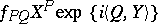in the Taylor–Fourier series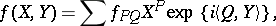(1)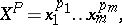whose indicatorsandsatisfy a linear relation as follows: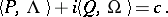(2)

Hereare constant coefficients,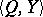is the scalar product ofand; the constants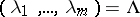and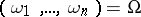are usually the eigenvalues and the basis of frequencies of a specific system of ordinary differential equations; the constantis independent ofandand it is defined by the role of the series (1) in the problem under analysis.

If in a linear system(3)

all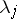are purely imaginary and in (2)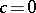, then the total resonance term of the series (1) coincides with the average of this series along the solutions of the system (3). A system of ordinary differential equations in a neighbourhood of invariant manifolds can be reduced to a normal form in which the series contains only resonance terms (see ). Thus, for a Hamiltonian system in a neighbourhood of a fixed point, the Hamiltonian function is reducible to the form (1) whereand (2) is fulfilled with, whenceis the vector of eigenvalues of the linearized system (see ). In this case, the terms,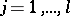, are sometimes called secular (for them (2) is fulfilled trivially), and the remaining terms of the series (1) for which (2) is fulfilled are called the resonance terms.

The separation of resonance terms, derived in problems with a small parameter, can often be based on a normal form (see ). For a point transformation with multipliersthe indices of the resonance terms of the series (1) withsatisfy the relation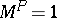; if one assumes that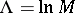and, then (2) is obtained with.